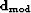Next: LSJIMP: Choice of Imaging Up: The LSJIMP Inverse problem Previous: The LSJIMP Inverse problem

## LSJIMP: Least-squares minimization

The LSJIMP method seeks to optimize the primary and multiple images,, by minimizing thenorm of the data residual, defined as the difference between the recorded data,, and the modeled data,[equation ()]:(4)
Minimization () is under-determined, for many choices of prestack imaging operator, which implies an infinite number of least-squares-optimal solutions. Practically speaking, this problem manifests itself as crosstalk leakage. If (for instance)contains residual first-order pegleg multiple energy, equation () will map this energy back into data space, at the position of a first-order multiple. Minimization () alone cannot distinguish between crosstalk and signal.

Of this infinity of possible's, we seek the one which not only fits the recorded data, but which also has minimum crosstalk leakage and maximum consistency between signal events on different images. In sectionI introduce model regularization operators to accomplish both goals.

After the minimization of equation (), theory dictates that the data residual should be independent and identically distributed (iid), or more intuitively, uncorrelated and evenly scaled. Correlated events in the residual imply that the forward model,, poorly models or fails to model events in the data. Examples of poor scaling might be the loss of reflection strength for deep reflectors, or empty traces. In general we compensate for a correlated or poorly scaled data residual by adding a residual weighting operator,:(5)
where strictly speaking,(6)
although in my implementation of LSJIMP, outlined in section, a far simpler choice foris used.Next: LSJIMP: Choice of Imaging Up: The LSJIMP Inverse problem Previous: The LSJIMP Inverse problem
Stanford Exploration Project
5/30/2004